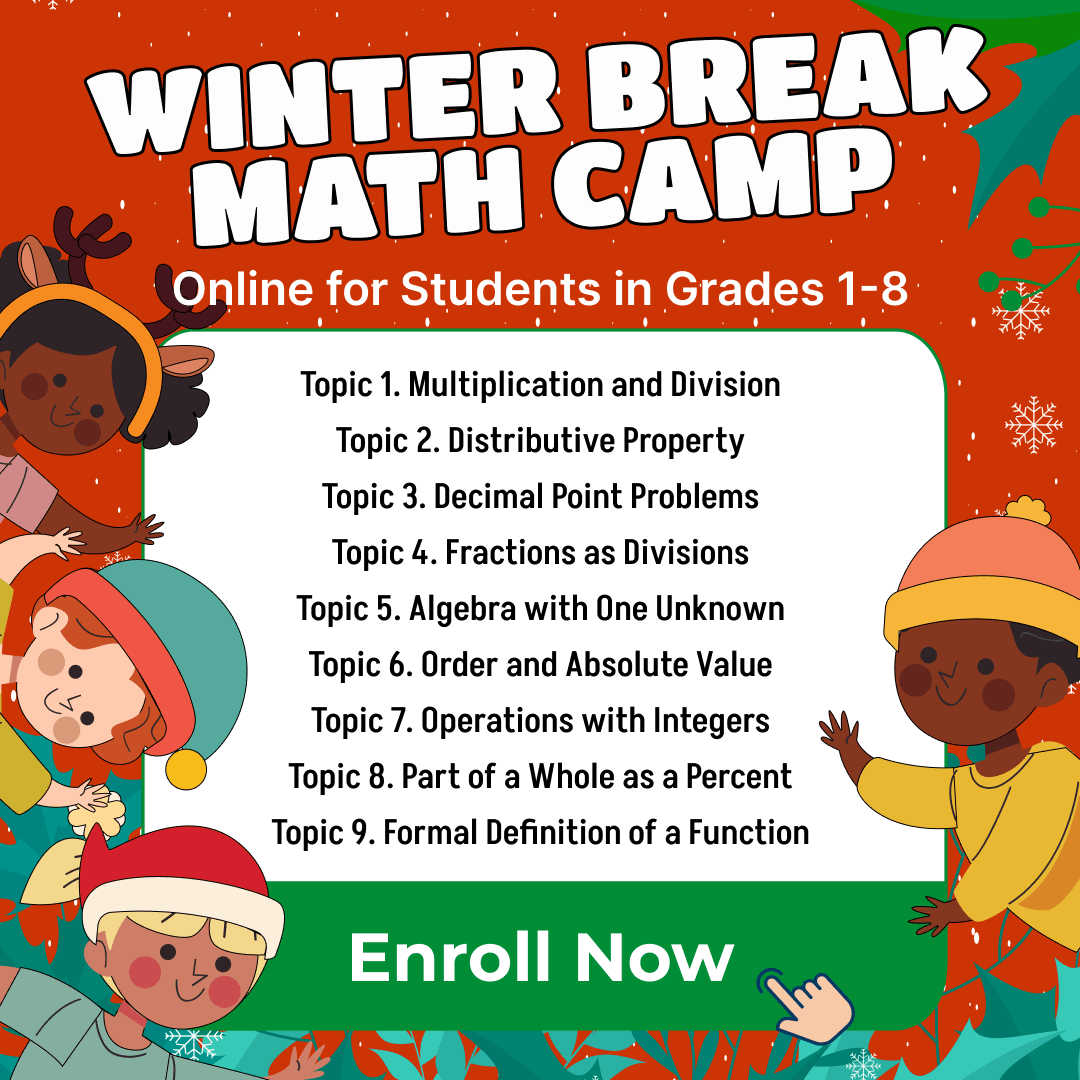# Numbers Math Practice Test for 3rd Grade – [Medium]

Numbers. They’re everywhere in our lives. From counting the pennies in our piggy banks to the number of stars in the sky. For 3rd graders, understanding numbers and their applications is not just a school task, it’s a rite of passage into a world of infinite possibilities. At Brighterly, we believe in making this journey both illuminating and fun!

## Whole Numbers and Place Value

When we talk about numbers, we often refer to whole numbers. But what are they? Whole numbers are simply the numbers we use to count, starting from zero and moving upwards. So, 1, 2, 3, and so forth.

The position of each digit in a number gives it its place value. For instance, in the number 204, 2 is in the hundreds place, 0 is in the tens place, and 4 is in the units place. Recognizing and understanding place values helps in performing operations like addition and subtraction efficiently.

## Fractions: Breaking it Down

Sometimes, a whole number just doesn’t cut it. Imagine splitting a pizza with a friend; you wouldn’t take 2 whole pizzas, but rather a fraction of it. Fractions represent parts of a whole.

The number above the fraction bar is the numerator and below is the denominator. If you ate 3 slices out of 8 from a pizza, then your fraction is 3/8.

## Introduction to Multiplication and Division

Multiplication is just a fast way of adding numbers. If you have 3 groups of 4 toys each, instead of adding 4+4+4, you can simply multiply 3×4 to get 12.

On the other hand, division is splitting something into equal parts. If you have 12 candies and want to share them with 4 friends equally, you’d divide 12 by 4, resulting in each friend getting 3 candies.

## Rounding Numbers: Keeping it Simple

Rounding numbers is making them simpler but still keeping their value close to what it was. Think of it as giving an estimate. If you have 47 marbles, and someone asks quickly, you might say “about 50” since 47 is closer to 50 than 40. That’s rounding!

This skill is very useful when dealing with larger numbers or when you need to make quick estimates.

## Prime and Composite Numbers

Numbers have personalities too! Prime numbers are numbers that can only be divided by 1 and themselves without leaving a remainder. Examples include 2, 3, 5, and so on.

Composite numbers, on the other hand, can be divided by other numbers as well. Like 4 can be divided by 2, and 6 can be divided by 2 and 3.

Numbers Practice Test for 3rd Grade

Get ready for math lessons with Brighterly! This medium-level test is crafted with precision and love, ensuring a balanced mix of fun and challenge.

1 / 15

Which of the following numbers is an even number?

2 / 15

How many sides does a triangle have?

3 / 15

If you round the number 74 to the nearest ten, what do you get?

4 / 15

Which of the following is NOT a prime number?

5 / 15

What is the sum of 45 and 30?

6 / 15

If you have 2 dozens of eggs, how many eggs do you have in total?

7 / 15

Which of the following fractions represents a half?

8 / 15

How many months are in a year?

9 / 15

What is the result of 63 divided by 7?

10 / 15

If you multiply 4 by 9, what is the result?

11 / 15

How many days are in a week?

12 / 15

Which number is between 10 and 12?

13 / 15

Which of the following numbers is the largest?

14 / 15

If you subtract 18 from 46, what do you get?

15 / 15

Which of the following is the smallest odd number?

0%
Poor Level
Weak math proficiency can lead to academic struggles, limited college, and career options, and diminished self-confidence.
Mediocre Level
Weak math proficiency can lead to academic struggles, limited college, and career options, and diminished self-confidence.
Needs Improvement
Start practicing math regularly to avoid your child`s math scores dropping to C or even D.
High Potential
It's important to continue building math proficiency to make sure your child outperforms peers at school.

Winter Break Math Camp• Boost Math Skills this Winter Break at our Camp, Perfect for Students in Grades 1-8!

Winter Break Math Camp
Enhance Math Skills with Our Program, Perfect for 1st-8th Graders, Aligned with School Curriculum!